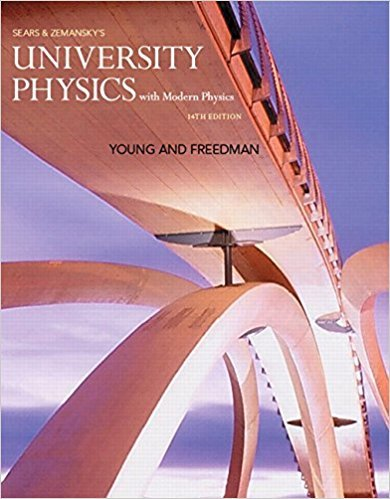×
×

# Solved: Does the speedometer of a car measure speed orISBN: 9780321973610 228

## Solution for problem Q2.1 Chapter 2

University Physics with Modern Physics (1) | 14th Edition

• Textbook Solutions
• 2901 Step-by-step solutions solved by professors and subject experts
• Get 24/7 help from StudySoup virtual teaching assistantsUniversity Physics with Modern Physics (1) | 14th Edition

4 5 1 327 Reviews
11
0
Problem Q2.1

Does the speedometer of a car measure speed or velocity? Explain

Step-by-Step Solution:
Step 1 of 3

Chapter 4 Two and Three Dimensional Motion The best way to work with multidimensional vectors is by using component form The position is written as:r=xi+yj+zk In this case x, y, and z would represent constants Displacement is shown as: ∆ r=∆ xi+∆ yj+∆zk...

Step 2 of 3

Step 3 of 3

##### ISBN: 9780321973610

Unlock Textbook Solution